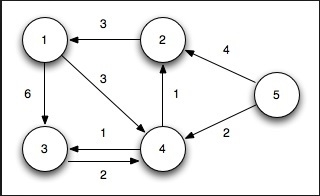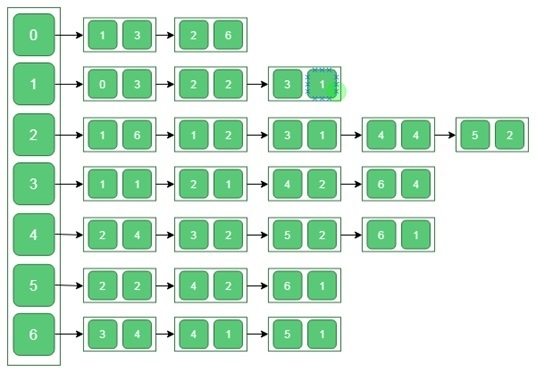# Dijkstra’s Algorithm for Adjacency List Representation

There is a given graph G(V, E) with its adjacency list representation, and a source vertex is also provided. Dijkstra’s algorithm to find the minimum shortest path between source vertex to any other vertex of the graph G.To Solve this problem, we will use two lists. One is to store vertices which have been considered as the shortest path tree, and another will hold the vertices which are not considered yet. In each phase of the algorithm, we find the unconsidered vertex and which has the minimum distance from the source.

Another list is used to hold the predecessor node. Using the predecessor node, we can find the path from source and destination.

The complexity of Dijkstra’s shortest path algorithm is O(E log V) as the graph is represented using adjacency list. Here the E is the number of edges, and V is Number of vertices.

## Input and Output

Input:
The adjacency list of the graph with the cost of each edge.Output:
0 to 1, Cost: 3 Previous: 0
0 to 2, Cost: 5 Previous: 1
0 to 3, Cost: 4 Previous: 1
0 to 4, Cost: 6 Previous: 3
0 to 5, Cost: 7 Previous: 2
0 to 6, Cost: 7 Previous: 4

## Algorithm

dijkstraShortestPath(g : Graph, dist, prev, start : node)

Input − The graph g, dist list to store distance, prev list for predecessor nodes, and start vertex.

Output − The shortest paths from start to all other vertices.

Begin
for all vertices u in (V - start) do
dist[u] := ∞
prev[u] := φ
done

set dist[start] = 0 and prev[start] := φ

for all node u in V do
insert u into queue ‘Q’.
done

while Q is not empty do
u := minimum element from Queue
delete u from Q
insert u into set S

for each node v adjacent with node u do
if dist[u]+cost(v) < dist[v] then
dist[v] := dist[u]+cost(v)
prev[v] := u
done
done
End

## Example

#include<iostream>
#include<set>
#include<list>
#include<algorithm>
using namespace std;

typedef struct nodes {
int dest;
int cost;
}node;

class Graph {
int n;
private:
void showList(int src, list<node> lt) {
list<node> :: iterator i;
node tempNode;

for(i = lt.begin(); i != lt.end(); i++) {
tempNode = *i;
cout << "(" << src << ")---("<<tempNode.dest << "|"<<tempNode.cost<<") ";
}
cout << endl;
}
public:
Graph() {
n = 0;
}

Graph(int nodeCount) {
n = nodeCount;
}

void addEdge(int source, int dest, int cost) {
node newNode;
newNode.dest = dest;
newNode.cost = cost;
}

void displayEdges() {
for(int i = 0; i<n; i++) {
showList(i, tempList);
}
}

friend void dijkstra(Graph g, int *dist, int *prev, int start);
};

void dijkstra(Graph g, int *dist, int *prev, int start) {
int n = g.n;

for(int u = 0; u<n; u++) {
dist[u] = 9999;   //set as infinity
prev[u] = -1;    //undefined previous
}

dist[start] = 0;   //distance of start is 0
set<int> S;       //create empty set S
list<int> Q;

for(int u = 0; u<n; u++) {
Q.push_back(u);    //add each node into queue
}

while(!Q.empty()) {
list<int> :: iterator i;
i = min_element(Q.begin(), Q.end());
int u = *i; //the minimum element from queue
Q.remove(u);
S.insert(u); //add u in the set
list<node> :: iterator it;

if((dist[u]+(it->cost)) < dist[it->dest]) { //relax (u,v)
dist[it->dest] = (dist[u]+(it->cost));
prev[it->dest] = u;
}
}
}
}

main() {
int n = 7;
Graph g(n);
int dist[n], prev[n];
int start = 0;

dijkstra(g, dist, prev, start);

for(int i = 0; i<n; i++)
if(i != start)
cout<<start<<" to "<<i<<", Cost: "<<dist[i]<<" Previous: "<<prev[i]<<endl;
}

## Output

0 to 1, Cost: 3 Previous: 0
0 to 2, Cost: 5 Previous: 1
0 to 3, Cost: 4 Previous: 1
0 to 4, Cost: 6 Previous: 3
0 to 5, Cost: 7 Previous: 2
0 to 6, Cost: 7 Previous: 4